Categories

## Processing: Roto Petey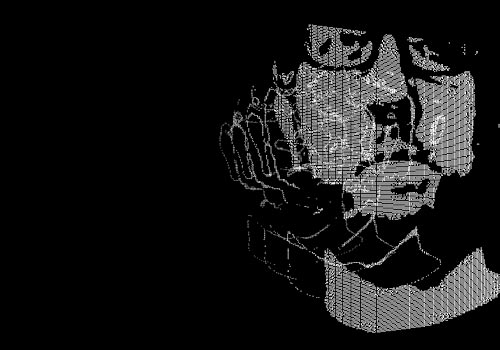Today’s Processing sketch isn’t really mine… It’s from the extrusion example on processing.org. It’s a cool demo showing how brightness of an image can map to height in 3D.

I took the code and put my own image into it. The image is from my profile pic, cropped a bit and posterized to 5 levels of grey.

This demo uses straight-up Java, not Javascript, because Processing.js doesn’t yet support all of what Processing does. (I was hoping to make a video of this but I’m having issues with the Processing MovieMaker code.)I find it useful to take code that someone else wrote, and start tweaking things, changing the values, etc. to see the outcome. I still find this code just a little confusing, but I’ll keep hacking at it to see what I can learn from it, and hope I can come up with something original myself.

```/*
* 20110507.pde
*/

PImage extrude;
int[][] values;
float angle = 0;

void setup() {
size(800, 600, P3D);
values = new int[extrude.width][extrude.height];
for (int y = 0; y < extrude.height; y++) {
for (int x = 0; x < extrude.width; x++) {
color pixel = extrude.get(x, y);
values[x][y] = int(brightness(pixel));
}
}
}

void draw() {
background(0);
angle += 0.01;

translate(width/2, 0, -128);
rotateY(angle);
translate(-extrude.width/2, 200, -180);

for (int y = 0; y < extrude.height; y++) {
for (int x = 0; x < extrude.width; x++) {
stroke(values[x][y]);
point(x, y, -values[x][y]);
}
}

}
```
Categories

## Processing: Mouse Droppings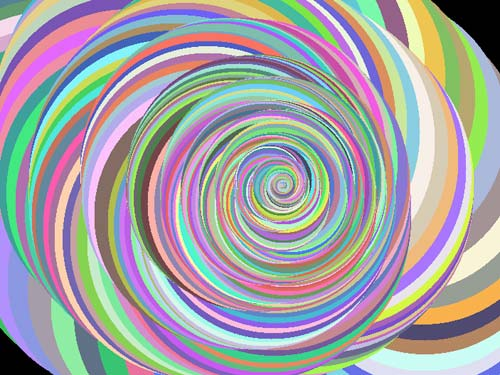OK, we’ve finally got a bit of interaction going on… This sketch presents a blank canvas, until you click the mouse button, and then you get a circle filled with some random color. As you moved the mouse (with the button held down) it’ll make more circles and before you know it, you’re drawing!

You can start over by pressing the ‘Q’ key, which will blank out the canvas. You can also adjust the size of the circle by using the up and down arrow keys.

```/*
* 20110506.pde
*/

void setup() {
size(800,600);
background(0);
}

color myColor = color(0,0,0);
int mySize = 50;

void draw() {
fill(myColor);
stroke(myColor);
strokeWeight(1);

if(keyPressed) {
if (key == 'q' || key == 'Q') {
background(0);
myColor = color(0,0,0);
mySize = 50;
}
if (key == CODED) {
if (keyCode == UP) {
mySize = mySize + 1;
}
else if (keyCode == DOWN) {
mySize = mySize - 1;
}
}
if (mySize < 1) {
mySize = 1;
}
if (mySize > 800) {
mySize = 800;
}
}

}

void mouseClicked() {
myColor = color(random(50,255), random(50,255), random(50,255));
}

void mouseDragged() {
myColor = color(random(50,255), random(50,255), random(50,255));
ellipse(mouseX, mouseY, mySize, mySize);
}
```

This is still a pretty minimal sketch. I did have to add in the upper and lower value limits on the size of the circle or things started to go in reverse if you held the key down too long.

Categories

## Processing: Flying Triangles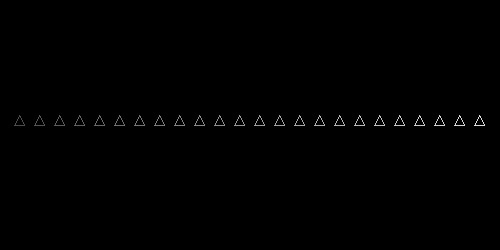Today’s Processing sketch features triangles of various shades flying upwards.

Pretty simple, eh? The original sketch this came from actually had just one triangle (which I’ll call a ‘spaceship’) which had the x-axis controlled by an Arduino with a potentiometer. This one doesn’t have that.

```/*
* 20110505.pde
*/

void setup() {
size(800, 200);
frameRate(30);
}

int yPos = 0;

void draw() {

background(0);
stroke(255);
fill(0);

yPos = yPos - 3;
if(yPos < 0) {
yPos = height;
}

for (int i = 20; i < 790; i = i+20) {
int yColor = i/3;
if (yColor > 255) {
yColor = 255;
}
stroke(yColor);
triangle(i, yPos, (i-5), (yPos+10), (i+5), (yPos+10));
}

}
```

I’d like to go back to the single ship again, and work on adding a starfield which moves past the ship as it maintains it’s vertical position on the screen.

Categories

## Processing: Square City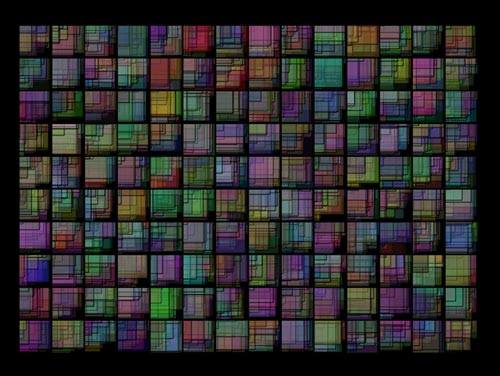For our latest installment of Processing Month, we’ve got a lot of squares, er, rectangles. They overlap. They are semi-transparent.

Another simple sketch, with randomness in it.

```/*
* 20110504.pde
*/

void setup() {
size(800, 600);
background(0);
}

float x = 50;
float y = 50;
int   c = 0;

void draw() {

fill(random(0,200),random(0,200),random(0,200), 70);
strokeWeight(0);
rect(x, y, random(1,50), random(1,50));

if (x > 650) {
x = 50;
y = y + 50;
}
else {
x = x + 50;
}

if (y > 500) {
y = 50;
if (c > 500) {
background(0);
c = 0;
}
c++;
}
}
```

It draws rectangles on top of rectangles on top of rectangles, and eventually clears the screen after enough iterations.

See any improvements you would make? Let me know…

Categories

## Processing: Overlapping Ovals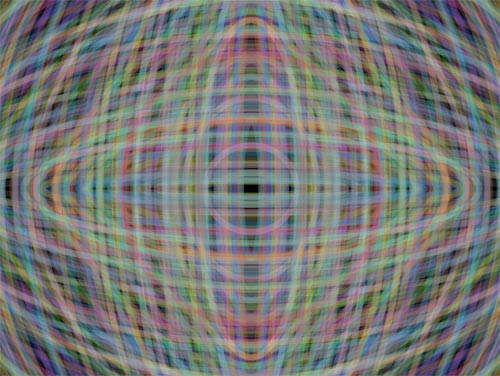I was unaware it was Processing Month, but I like the idea… so I’m going to try to kick out some sketches this month.

I’ll start off slow and (hopefully) see some improvement by the end of the month. This sketch is called “Overlapping Ovals” and you should be able to see it in action here if you’ve got a canvas-capable browser. (We’re using Processing.js to do the magic. I’m not sure that’s the best way to do this, but we’ll find out.)

```/*
* 20110503.pde
*/

void setup() {
size(800, 600);
background(0);
}

int c = 1;

void draw() {

noFill();

float rCol = random(50,240);
float gCol = random(50,240);
float bCol = random(50,240);
stroke(rCol,gCol,bCol,50);
strokeWeight(10);

float wNum = random(20,1200);
float hNum = random(20,1200);
ellipse((800/2), (600/2), wNum, hNum);

if (c > 1000) {
background(0);
c = 1;
}

c++;

}
```

It basically draws 1000 randomly sized and colored ellipse on the screen, then clears the canvas, and does it again.

Again, I’m still a bit of a Processing newbie, so be kind, and hope that things get better as we go along. If you see something that seems all wrong, call it out so I can learn and improve it.